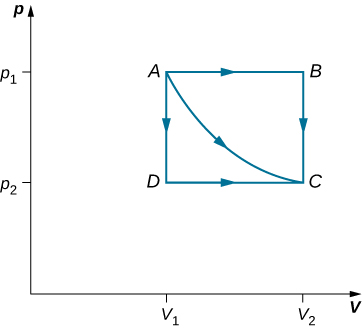# 3.2 Work, heat, and internal energy  (Page 2/6)

 Page 2 / 6
$W={\int }_{{V}_{1}}^{{V}_{2}}pdV={\int }_{{V}_{1}}^{{V}_{2}}\left(\frac{nRT}{V}\right)\phantom{\rule{0.2em}{0ex}}dV.$The paths ABC , AC , and ADC represent three different quasi-static transitions between the equilibrium states A and C .

The expansion is isothermal, so T remains constant over the entire process. Since n and R are also constant, the only variable in the integrand is V , so the work done by an ideal gas in an isothermal process is

$W=nRT{\int }_{{V}_{1}}^{{V}_{2}}\frac{dV}{V}=nRT\text{ln}\frac{{V}_{2}}{{V}_{1}}.$

Notice that if ${V}_{2}>{V}_{1}$ (expansion), W is positive, as expected.

The straight lines from A to B and then from B to C represent a different process. Here, a gas at a pressure ${p}_{1}$ first expands isobarically (constant pressure) and quasi-statically from ${V}_{1}\phantom{\rule{0.2em}{0ex}}\text{to}\phantom{\rule{0.2em}{0ex}}{V}_{2}$ , after which it cools quasi-statically at the constant volume ${V}_{2}$ until its pressure drops to ${p}_{2}$ . From A to B , the pressure is constant at p , so the work over this part of the path is

$W={\int }_{{V}_{1}}^{{V}_{2}}pdV={p}_{1}{\int }_{{V}_{1}}^{{V}_{2}}dV={p}_{1}\left({V}_{2}-{V}_{1}\right).$

From B to C , there is no change in volume and therefore no work is done. The net work over the path ABC is then

$W={p}_{1}\left({V}_{2}-{V}_{1}\right)+0={p}_{1}\left({V}_{2}-{V}_{1}\right).$

A comparison of the expressions for the work done by the gas in the two processes of [link] shows that they are quite different. This illustrates a very important property of thermodynamic work: It is path dependent . We cannot determine the work done by a system as it goes from one equilibrium state to another unless we know its thermodynamic path. Different values of the work are associated with different paths.

## Isothermal expansion of a van der waals gas

Studies of a van der Waals gas require an adjustment to the ideal gas law that takes into consideration that gas molecules have a definite volume (see The Kinetic Theory of Gases ). One mole of a van der Waals gas has an equation of state

$\left(p+\frac{a}{{V}^{2}}\right)\left(V-b\right)=RT,$

where a and b are two parameters for a specific gas. Suppose the gas expands isothermally and quasi-statically from volume ${V}_{1}$ to volume ${V}_{2}.$ How much work is done by the gas during the expansion?

## Strategy

Because the equation of state is given, we can use [link] to express the pressure in terms of V and T . Furthermore, temperature T is a constant under the isothermal condition, so V becomes the only changing variable under the integral.

## Solution

To evaluate this integral, we must express p as a function of V . From the given equation of state, the gas pressure is

$p=\frac{RT}{V-b}-\frac{a}{{V}^{2}}.$

Because T is constant under the isothermal condition, the work done by 1 mol of a van der Waals gas in expanding from a volume ${V}_{1}$ to a volume ${V}_{2}$ is thus

$\begin{array}{cc}\hfill W& =\underset{{V}_{1}}{\overset{{V}_{2}}{\int }}\left(\frac{RT}{V-b}-\frac{a}{{V}^{2}}\right)={|RT\text{ln}\left(V-b\right)+\frac{a}{V}|}_{{V}_{1}}^{{V}_{2}}\hfill \\ & =RT\text{ln}\left(\frac{{V}_{2}-b}{{V}_{1}-b}\right)+a\left(\frac{1}{{V}_{2}}-\frac{1}{{V}_{1}}\right).\hfill \end{array}$

## Significance

By taking into account the volume of molecules, the expression for work is much more complex. If, however, we set $a=0$ and $b=0,$ we see that the expression for work matches exactly the work done by an isothermal process for one mole of an ideal gas.

Check Your Understanding How much work is done by the gas, as given in [link] , when it expands quasi-statically along the path ADC ?

${p}_{2}\left({V}_{2}-{V}_{1}\right)$

## Internal energy

The internal energy     ${E}_{\text{int}}$ of a thermodynamic system is, by definition, the sum of the mechanical energies of all the molecules or entities in the system. If the kinetic and potential energies of molecule i are ${K}_{i}$ and ${U}_{i},$ respectively, then the internal energy of the system is the average of the total mechanical energy of all the entities:

what are waves
In physics, mathematics, and related fields, a wave is a propagating dynamic disturbance (change from equilibrium) of one or more quantities
Discuss how would orient a planar surface of area A in a uniform electric field of magnitude E0 to obtain (a) the maximum flux and (b) the minimum flux through the area.
I'm just doing the first 3 with this message. but thankyou for the time your obviously intending to support us with. viva la accumulation
Marcel
Find the net capacitance of the combination of series and parallel capacitors shown belo
what is ohm?
calculate ideal gas pressure of 0.300mol,v=2L T=40°c
what is principle of superposition
what are questions that are likely to come out during exam
what is electricity
watt is electricity.
electricity ka full definition with formula
Jyoti
If a point charge is released from rest in a uniform electric field will it follow a field line? Will it do so if the electric field is not uniform?
Maxwell's stress tensor is
Yes
doris
neither vector nor scalar
Anil
if 6.0×10^13 electrons are placed on a metal sphere of charge 9.0micro Coulombs, what is the net charge on the sphere
18.51micro Coulombs
ASHOK
Is it possible to find the magnetic field of a circular loop at the centre by using ampere's law?
Is it possible to find the magnetic field of a circular loop at it's centre?
yes
Brother
The density of a gas of relative molecular mass 28 at a certain temperature is 0.90 K kgmcube.The root mean square speed of the gas molecules at that temperature is 602ms.Assuming that the rate of diffusion of a gas in inversely proportional to the square root of its density,calculate the density of
A hot liquid at 80degree Celsius is added to 600g of the same liquid originally at 10 degree Celsius. when the mixture reaches 30 degree Celsius, what will be the total mass of the liquid?
Under which topic
doris
what is electrostatics
Study of charges which are at rest
himanshu

#### Get Jobilize Job Search Mobile App in your pocket Now!ByBy Laurence BailenBy OpenStaxBy Rohini AjayBy OpenStaxBy Jessica CollettBy LaToya TrowersBy Mary MateraBy Janet ForresterBy Jazzycazz Jackson